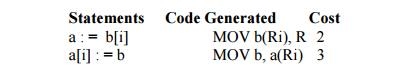Home | | Compiler Design | A Simple Code Generator

# A Simple Code Generator

If the name in a register is no longer needed, then we remove the name from the register and the register can be used to store some other names.

NEXT-USE INFORMATION

• If the name in a register is no longer needed, then we remove the name from the register and the register can be used to store some other names.

Input: Basic block B of three-address statements

Output: At each statement i: x= y op z, we attach to i the liveliness and next-uses of x, y and z.

Method: We start at the last statement of B and scan backwards.

1. Attach to statement i the information currently found in the symbol table regarding the next-use and liveliness of x, y and z.

2.   In the symbol table, set x to “not live” and “no next use”.A SIMPLE CODE GENERATOR

• A code generator generates target code for a sequence of three- address statements and

effectively uses registers to store operands of the statements.

• For example: consider the three-address statement a := b+c It can have the following sequence of codes:

ADD Rj, Ri Cost = 1

(or)

ADD c, Ri Cost = 2

(or)

MOV c, Rj Cost = 3

• A register descriptor is used to keep track of what is currently in each registers. The register

descriptors show that initially all the registers are empty.

• An address descriptor stores the location where the current value of the name can be found at run time.

A code-generation algorithm:

The algorithm takes as input a sequence of three-address statements constituting a basic block. For each three-address statement of the form x : = y op z, perform the following actions:

1.   Invoke a function getreg to determine the location L where the result of the computation y op z should be stored.

2.     Consult the address descriptor for y to determine y’, the current location of y. Prefer the register for y’ if the value of y is currently both in memory and a register. If the value of y is not already in L, generate the instruction MOV y’ , L to place a copy of y in L.

3.    Generate the instruction OP z’ , L where z’ is a current location of z. Prefer a register to a memory location if z is in both. Update the address descriptor of x to indicate that x is in location L. If x is in L, update its descriptor and remove x from all other descriptors.

4. If the current values of y or z have no next uses, are not live on exit from the block, and are in registers, alter the register descriptor to indicate that, after execution of x : = y op z , those registers will no longer contain y or z

Generating Code for Assignment Statements:

• The assignment d : = (a-b) + (a-c) + (a-c) might be translated into the following three-address code sequence:

Code sequence for the example is:Generating Code for Indexed Assignments

The table shows the code sequences generated for the indexed assignmen a:= b[ i ] and a[ i ]:= bGenerating Code for Pointer Assignments

The table shows the code sequences generated for the pointer assignments a : = *p and *p : = aif x < 0 goto z ADD z, R0

MOV R0,x

CJ< z

Study Material, Lecturing Notes, Assignment, Reference, Wiki description explanation, brief detail
Principles of Compiler Design : Code Generation : A Simple Code Generator |